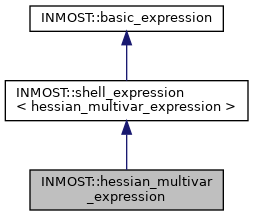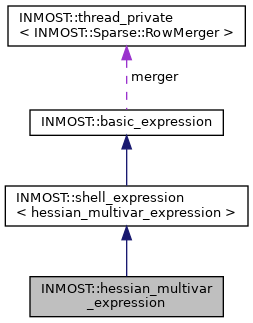INMOST A toolkit for distributed mathematical modeling
INMOST::hessian_multivar_expression Class Reference

A class that represents a variable with multiple first order and second order variations. More...

`#include <inmost_expression.h>`

Inheritance diagram for INMOST::hessian_multivar_expression:[legend]
Collaboration diagram for INMOST::hessian_multivar_expression:[legend]

## Public Member Functions

void swap (hessian_multivar_expression &b)

hessian_multivar_expression ()
Sets zero value and no first or second order variations.

hessian_multivar_expression (INMOST_DATA_REAL_TYPE pvalue)
Sets value and no first or second order variations.

hessian_multivar_expression (const hessian_multivar_expression &other)
Copy value and all first and second order variations from hessian_variable.

hessian_multivar_expression (INMOST_DATA_REAL_TYPE pvalue, Sparse::Row &pentries)
Copy value and all first variations from variable, no second order variations. More...

hessian_multivar_expression (INMOST_DATA_REAL_TYPE pvalue, Sparse::Row &pentries, Sparse::HessianRow &phentries)
Sets value and all first and second order variations.

hessian_multivar_expression (INMOST_DATA_REAL_TYPE pvalue, INMOST_DATA_ENUM_TYPE pindex, INMOST_DATA_REAL_TYPE pdmult=1.0)
Sets value and it's variation index.

hessian_multivar_expression (const basic_expression &expr)
Evaluates argument expression to get the value and all variations of variable.

__INLINE INMOST_DATA_REAL_TYPE GetValue () const

__INLINE void SetValue (INMOST_DATA_REAL_TYPE val)

__INLINE void GetJacobian (INMOST_DATA_REAL_TYPE mult, Sparse::RowMerger &r) const

__INLINE void GetJacobian (INMOST_DATA_REAL_TYPE mult, Sparse::Row &r) const

__INLINE void GetHessian (INMOST_DATA_REAL_TYPE multJ, Sparse::Row &J, INMOST_DATA_REAL_TYPE multH, Sparse::HessianRow &H) const

__INLINE INMOST_DATA_ENUM_TYPE GetCount () const

__INLINE multivar_expression GetVariable (INMOST_DATA_ENUM_TYPE index)

__INLINE hessian_multivar_expressionoperator= (INMOST_DATA_REAL_TYPE pvalue)

__INLINE hessian_multivar_expressionoperator= (hessian_multivar_expression const &other)

__INLINE Sparse::RowGetRow ()

__INLINE Sparse::HessianRowGetHessianRow ()

__INLINE const Sparse::RowGetRow () const

__INLINE INMOST_DATA_REAL_TYPE GetDerivative (INMOST_DATA_ENUM_TYPE index) const

__INLINE const Sparse::HessianRowGetHessianRow () const

__INLINE hessian_multivar_expressionoperator+= (basic_expression const &expr)

__INLINE hessian_multivar_expressionoperator-= (basic_expression const &expr)

__INLINE hessian_multivar_expressionoperator*= (basic_expression const &expr)

__INLINE hessian_multivar_expressionoperator/= (basic_expression const &expr)

__INLINE hessian_multivar_expressionoperator+= (INMOST_DATA_REAL_TYPE right)

__INLINE hessian_multivar_expressionoperator-= (INMOST_DATA_REAL_TYPE right)

__INLINE hessian_multivar_expressionoperator*= (INMOST_DATA_REAL_TYPE right)

__INLINE hessian_multivar_expressionoperator/= (INMOST_DATA_REAL_TYPE right)

bool check_nans () const

bool check_infs () constPublic Member Functions inherited from INMOST::shell_expression< hessian_multivar_expression >
operator hessian_multivar_expression & ()

operator const hessian_multivar_expression & () const

## Friends

class hessian_multivar_expression_referenceStatic Protected Attributes inherited from INMOST::basic_expression

## Detailed Description

A class that represents a variable with multiple first order and second order variations.

Short type name is hessian_variable.

Definition at line 407 of file inmost_expression.h.

## ◆ hessian_multivar_expression()

 INMOST::hessian_multivar_expression::hessian_multivar_expression ( INMOST_DATA_REAL_TYPE pvalue, Sparse::Row & pentries )
inline

Copy value and all first variations from variable, no second order variations.

Sets value and all first variations, no second order variations.

Definition at line 428 of file inmost_expression.h.

The documentation for this class was generated from the following file: